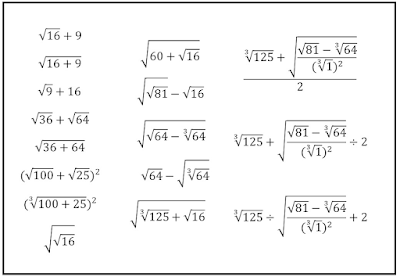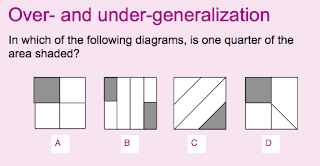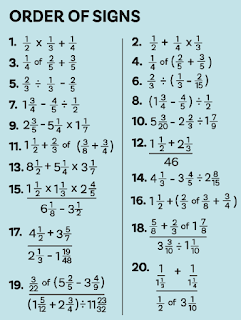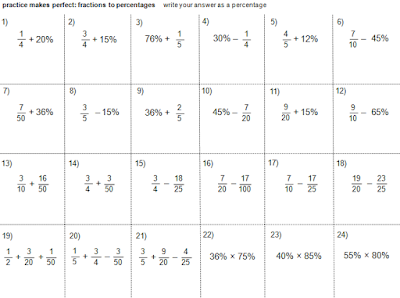## Pages

### NumberThis page lists recommended resources for teaching number topics at Key Stage 3/4.

Huge thanks to all individuals and organisations who share teaching resources.

Quick links: Negatives  |  Place Value  |  Addition & Subtraction  |  Multiplication & Division  |  Order of Operations  |  Decimals  |  Rounding  |  Estimation  |  Calculator Skills  |  Fractions  |  Percentages  |  Growth & Decay  |  Ratio & Proportion  |  Factors, Multiples and Number Properties  |  Recurring Decimals  |  Indices  |  Surds  |  Standard Form

Negative NumbersMagic Squares - MathsPad
Place ValueMissing Numbers
Multiplication and DivisionRoots task from Dan Draper

Order of Operations   (see my topics in depth pack)Operation Countdown by @taylorda01
Decimals

Rounding@OxfordEdMaths

Estimation

Calculator Skills

FractionsHinge question from Dylan William
ArithmeticFDP

PercentagesFrom @MathsMastery

Interest and repeated percentage change below.Fractions to percentages by Don Steward

Growth and Decay and Interest

Ratio and Proportion
Ratio

Proportion

Proportion formulae

Factors, Multiples and Number PropertiesSquares, cubes and roots (SMART worksheet 2095)

Recurring DecimalsGiantburger - Mathematics Assessment Project